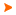Podaci o VU Početna stranicaArhiva nastavnih programaArhiva ak. kalendaraMathematics IV A
 Abbreviation: MAT IV-A Load: 15(L) + 15(E) + 0(LE) + 0(CE) + 0(PEE) + 0(FE) + 0(S) + 0(DE) + 0(P) + 0(FLE) + 0()
 Lecturers in charge: prof. dr. sc. Sanja Singer Lecturers: Ivan Čeh ( Exercises ) Course description: Course objectives: To understand concepts of the numerical mathematics and scientific computing. Usage of the numerical methods with emphasys on possible errors in obtained results.Enrolment requirements and required entry competences for the course: Passed exam Matematika II.Student responsibilities: Get 15% of the midterm exam to get the right to go to the written exam.Grading and evaluation of student work over the course of instruction and at a final exam: Passing a midterm exam (45%) is equivalent to a written exam.Methods of monitoring quality that ensure acquisition of exit competences: Written exam.Upon successful completion of the course, students will be able to (learning outcomes): After the final exam, students will be able to describe the basics of the numerical methods, and find the solustion the simple numerical problems. Especially, they will be able to describe errors of the computer arithmetics.Lectures 1. Introduction to numerical mathematics and scientific computing.2. IEEE arithmetics. Errors.3. Direct methods for solution of linear systems. Gaussian eliminations.4. LR factorization. Pivoting. Condition number.5. Introduction to approximations. Types of approximations.6. Polynomial interpolation. Egzistence and uniqueness of the interpolationg polynomial.7. Lagrange"s form of the interpolating polynomial. Interpolation error.8. Newton"s form of the interpolating polynomial. Divided differences.9. Method of least squares.10. Numerical integration. NewtonCotes i Gaussian formulae.11. Trapezoidal and Simpson"s rule. Midpoint rule.12. Numerical solution of a nonlinear equation. Method of bisection.13. Newton"s method for nonlinear equations. Convergence.14. Methods for the initial value problem ODE. RK methods and multistep methods.15. Stiff differential equations.Exercises 1. Introduction to numerical mathematics and scientific computing.2. IEEE arithmetics. Errors.3. Direct methods for solution of linear systems. Gaussian eliminations.4. LR factorization. Pivoting. Condition number.5. Introduction to approximations. Types of approximations.6. Polynomial interpolation. Egzistence and uniqueness of the interpolationg polynomial.7. Lagrange"s form of the interpolating polynomial. Interpolation error.8. Newton"s form of the interpolating polynomial. Divided differences.9. Method of least squares.10. Numerical integration. NewtonCotes i Gaussian formulae.11. Trapezoidal and Simpson"s rule. Midpoint rule..12. Numerical solution of a nonlinear equation. Method of bisection.13. Newton"s method for nonlinear equations. Convergence.14. Methods for the initial value problem ODE. RK methods and multistep methods.15. Stiff differential equations.
 Lecture languages: hr
 Compulsory literature: 1. Pisani materijali za predmet Matematika 3 i 4, dostupni na e-ucenje.fsb.hr.
 Recommended literature: 2. Ivo Alfirević i dr., Inženjerski priručnik 1, Školska knjiga, Zagreb, 1996.
 Prerequisit for enrollment: Passed : Mathematics IILegend L - Lectures FLE - Practical foreign language exercises - E - Exercises LE - Laboratory exercises CE - Project laboratory PEE - Physical education excercises FE - Field exercises S - Seminar DE - Design exercises P - Practicum * - Not graded| Početna stranica | Visoka učilišta | Korisničke stranice | | Na vrh | Copyright (c) 2006. Ministarstva znanosti, obrazovanja i športa. Sva prava zadržana. Programska podrška (c) 2006. Fakultet elektrotehnike i računarstva. Oblikovanje(c) 2006. Listopad Web Studio. Posljednja izmjena 2019-06-07